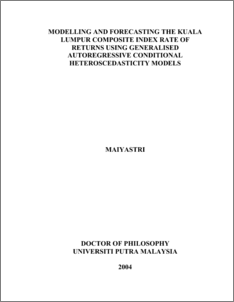# Modelling and Forecasting the Kuala Lumpur Composite Index Rate of Returns Using Generalised Autoregressive Conditional Heteroscedasticity Models

## Citation

Abdul Muthalib, Maiyastri (2004) Modelling and Forecasting the Kuala Lumpur Composite Index Rate of Returns Using Generalised Autoregressive Conditional Heteroscedasticity Models. PhD thesis, Universiti Putra Malaysia.

## Abstract

The work in this thesis is concerned with the modelling and forecasting of the KLCI’s returns with a ‘complete’ technique. The selection of the model for estimation is not only based on the value of the goodness of fit test, but also on the test of the stability of parameters obtained, the checking of the characteristic of the standardized residual such as the ARCH-LM test, Ljung-Box test, asymmetry test (SB, NSB, and PSB test). Its distribution is examined by qq-plot. The forecasting is carried out by using the multi-step-ahead forecast. This study also proposes the PCA(Principal Component Analysis) as an alternative method to compare the performance of several GARCH models. It is found that this method has a clear edge over its rival because PCA uses actual values of the goodness of fit test criteria (LogL, SBC, and AIC in estimation and RMSE, MAE, AMAPE and MAPE in forecasting) and hence the inability to specify exactly the relative positions of each of the competing models as faced by the ranking method may be overcome. Another plus point is that, this method enables models to be classified into several distinct groups ordered in such a way that each group is made up of models with about the same level of fitting and forecasting abilities. The characteristics of the numerous tests in modelling for GARCH have been checked by Monte Carlo simulation. The tests are the ARCH-LM test, the SB, NSB and PSB tests, the parameters constancy test and the ARCH remaining test (to test whether the standardized residual still contain signs of conditional heteroscedasticity). The results show that the ARCH remaining test is inconsistent, but the other tests are accurate and stable for all experiments. Since the volatility of the KLCI’s returns series is non-uniform, the data is split into three periods of time. In the beginning, the division of the data is based on the plot of the returns, but for the later part, it is based on the distribution of the returns. Methods for correcting the outliers and splitting the heterogeneous data are proposed. The EM algorithm is applied to split the heterogeneous data, and the estimated parameters are used to correct the outlying data using the Mahalanobis Distance. The last part of the thesis is concerned with the validation of the model obtained for fitting the GARCH model for the new data set (January 2001-April 2004 or Period IV). It is found that the pattern of the new data set resembles of Period III, and both models are also the same. Finally, the model selected for Period IV is used to determine the VaR (Value at Risk) of the KLCI. The VaR at the 5% level for next 5 trading days (the next week) starting from the forecast origin on the 30th April 2004 is RM 35,492.55, which means that if someone invest RM 1 million in the KLSE, and if some extraordinary event happens, the maximum loss incurred for the next 5 trading days (starting from the forecast origin on the 30th April 2004) with 95% probability is RM 35,492.55.Preview
PDF
549765_fs_2004_17_abstrak_je__dh_pdf_.pdfView Item